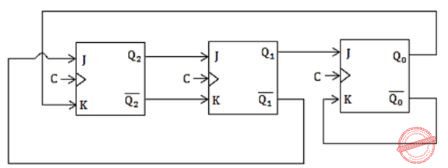## Flip-Flops

 Question 1The above synchronous sequential circuit built using JK flip-flops is initialized with Q2Q1Q0 = 000. The state sequence for this circuit for the next 3 clock cycles is

 A 001, 010, 011 B 111, 110, 101 C 100, 110, 111 D 100, 011, 001
Digital-Logic-Design       Flip-Flops       GATE 2014 [Set-3]
Question 1 Explanation:Question 2

The number of flip-flops required to construct a binary modulo N counter is __________.

 A ⌈log2 N⌉
Digital-Logic-Design       Flip-Flops       GATE 1994
Question 2 Explanation:
For mod-N counter we need ⌈log2 N⌉ flip flops.
 Question 3

Find the contents of the flip-flop Q2, Q1 and Q0 in the circuit of figure, after giving four clock pulses to the clock terminal. Assume Q2Q1Q0 = 000 initially.A Theory Explanation.
Digital-Logic-Design       Flip-flops       GATE 1994
There are 3 questions to complete.

Register Now